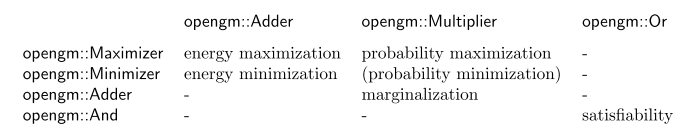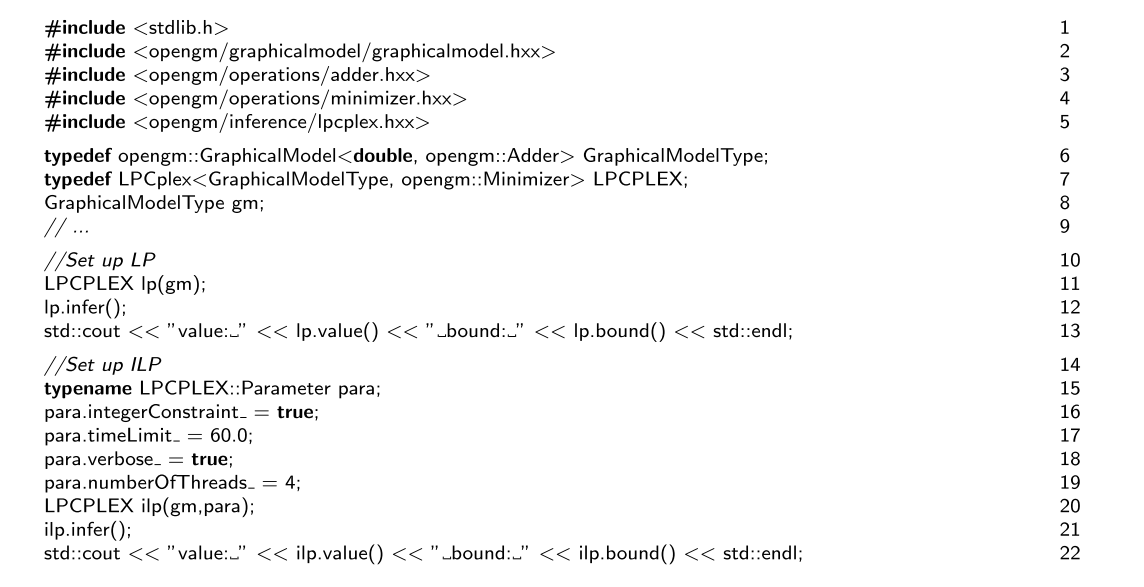Today I will be discussing some of the ways in which inference algorithms especially the Linear Programming ones work in OpenGM.
So there are many ways to solve an energy minimization problem.
From the table below we can get an idea about the different accumulative operations used to do inference:That is, if we need to solve for energy minimization problem, we have to go for a combination of opengm::Adder and opengm::Minimizer in OpenGM to bring the same effect.

Along with this, we have to choose one Inference algorithm. From OpenGM we get the list of available inference algorithms as below:

1. Message Passing
1. Belief Propogation
2. TRBP
2. TRW-S
3. Dynamic Programming
4. Dual Decomposition
1. Using Sub-gradient Methods
2. Using Bundle Methods
5. A* Search
6. (Integer) Linear Programming
7. Graph Cuts
8. QPBO
9. Move Making
1. Expansion
2. Swap
3. Iterative Conditional Modes(ICM)
4. Lazy Flipper
5. LOC
10. Sampling
1. Gibbs
2. Swendsen-Wang
11. Wrappers around other libraries
1. libDAI
2. MRF-LIB
12. BruteForce Search

We will be concentrating here on the ILP Relaxation problem. Lets see how it goes!

Any energy minimization problem can be formulated as an (integer) linear problem. We can also sufficiently solve the problem by considering a relaxation of the original problem by relaxing the system of imequlities.

The corresponding parameter class can take many optional parameters which can be used to tune the solver. The most important are the flags

• to switch to integer mode integerConstraint_
• the number of threads that should be used numberOfThreads_
• the verbose flag verbose_ that switches the CPLEX output on
• the timeLimit_ that stops the method after N seconds realtime

A sample is shown below: